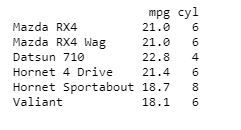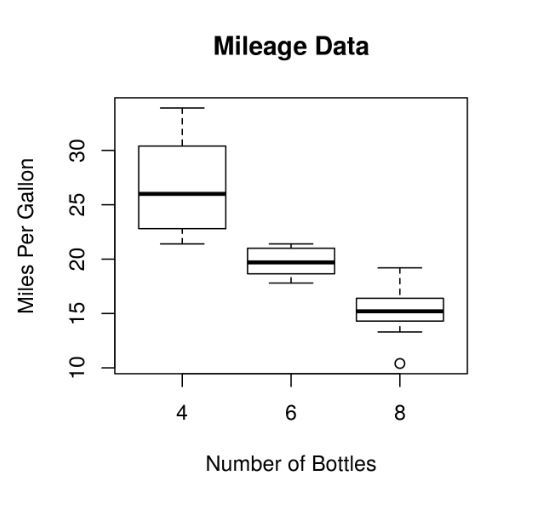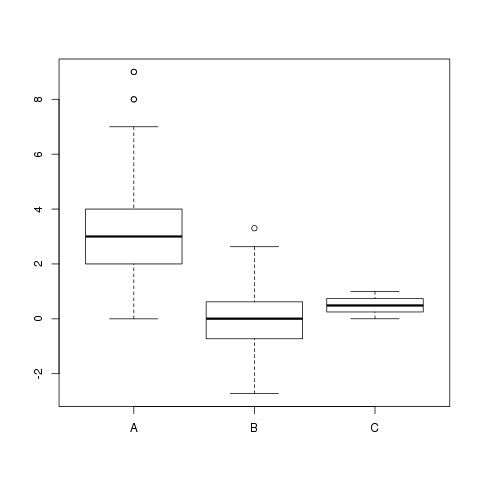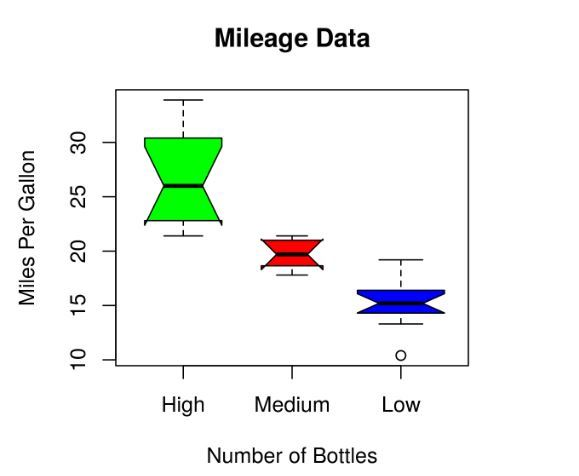GFG App
Open AppBrowser
Continue

A box graph is a chart that is used to display information in the form of distribution by drawing boxplots for each of them. This distribution of data based on five sets (minimum, first quartile, median, third quartile, maximum).

## Boxplots in R Programming Language

Boxplots are created in R by using the boxplot() function.

Syntax: boxplot(x, data, notch, varwidth, names, main)

Parameters:

• x: This parameter sets as a vector or a formula.
• data: This parameter sets the data frame.
• notch: This parameter is the label for horizontal axis.
• varwidth: This parameter is a logical value. Set as true to draw width of the box proportionate to the sample size.
• main: This parameter is the title of the chart.
• names: This parameter are the group labels that will be showed under each boxplot.

## Creating a Dataset

To understand how we can create a boxplot:

• We use the data set “mtcars”.
• Let’s look at the columns “mpg” and “cyl” in mtcars.

## R

 `input <- mtcars[, ``c``(``'mpg'``, ``'cyl'``)]` `print``(``head``(input))`

Output:### Creating the Boxplot

Creating the Boxplot graph.

• Take the parameters which are required to make boxplot.
• Now we draw a graph for the relation between “mpg” and “cyl”.

## R

 `# Plot the chart.` `boxplot``(mpg ~ cyl, data = mtcars,` `        ``xlab = ``"Number of Cylinders"``,` `        ``ylab = ``"Miles Per Gallon"``,` `        ``main = ``"Mileage Data"``)`

Output:### Multiple Boxplot

Here we are creating multiple boxplots. The individual data for which a boxplot representation is required is based on the function.

## R

 `set.seed``(20000)                                        ` `data <- ``data.frame``( A = ``rpois``(900, 3),` `                    ``B = ``rnorm``(900),` `                    ``C = ``runif``(900)` `                    `  `                    `  `)`   `# Applying boxplot function` `boxplot``(data)`

Output:### Boxplot using notch

To draw a boxplot using a notch:

• With the help of notch, we can find out how the medians of different data groups match with each other.
• We are using xlab as “Quantity of Cylinders” and ylab as “Miles Per Gallon”.

## Python3

 `# Plot the chart.` `boxplot(mpg ~ cyl, data ``=` `mtcars,` `        ``xlab ``=` `"Number of Cylinders"``,` `        ``ylab ``=` `"Miles Per Gallon"``,` `        ``main ``=` `"Mileage Data"``,` `        ``notch ``=` `TRUE,` `        ``varwidth ``=` `TRUE,` `        ``col ``=` `c(``"green"``, ``"red"``, ``"blue"``),` `        ``names ``=` `c(``"High"``, ``"Medium"``, ``"Low"``)` `        ``)`

Output:My Personal Notes arrow_drop_up